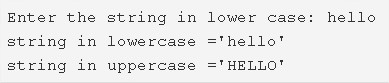# C Program To Convert Lowercase String To Uppercase | 4 Ways

C program to convert a lowercase string to uppercase – In this article, we will brief in on the multiple ways to convert a lowercase string to uppercase in C programming.

Suitable examples and sample programs have also been added so that you can understand the whole thing very clearly. The compiler has also been added with which you can execute it yourself.

In this piece, the ways used are as follows:

• Using Standard Method
• Using Function
• Using Recursion
• Using String Library Function

A string is nothing but an array of characters. The value of a string is determined by the terminating character. Its value is considered to be 0.As you can see in the image uploaded above, firstly, we need to enter a particular string in lowercase which we want to convert to uppercase.

The string entered here is: hello

Hence, the lowercase string, in this case, will be: HELLO

Thus, the different methods to convert a specific lowercase string to uppercase are as follows:

## Using Standard Method

1) Read the entered string which is in the lower case using gets(s).

2) Check the each ASCII value of the string is in the range between 97 to 122(lowercase alphabets range).If the character is in lower case then subtract 32 from its ASCII value, then the character will be converted into upper case.

3) After all iterations of for loop print the string which is in upper case.

Output:

### Using Function

1. The main() calls the stringuppercase() by passing the string as an argument.

2) The function stringuppercase() checks whether the character is in upper case or not by checking its ASCII value. If any character is in lower case(the range is between 97 to 122) then subtract 32 from its ASCII value, then it will be converted into upper case. The function repeats this until all iterations of for loop.

3) The main() function prints the upper case string.

Output:

#### Using Recursion

1. If the character is available in the string then the function stringuppercase() checks the character s[i] is lower case or upper case by checking its ASCII value.

2) If the character is lower case alphabet then subtract 32 from its ASCII value as s[i++]-=32 and the upper case alphabet will be placed into s[i++].

3) The function calls itself by passing modified string s as an argument. It calls recursively until there is no alphabet is available to check the ASCII.

4) The main() function prints the upper case string.

Output:
##### Using String Library Function
1. The strupr() is the library function available at string.h header file which converts lower case string into upper case string.
Output:
x

## C Program To Check If Alphabet, Digit or Special Character | C Programs

C program to check for an alphabet, digit or special character – In this article, ...